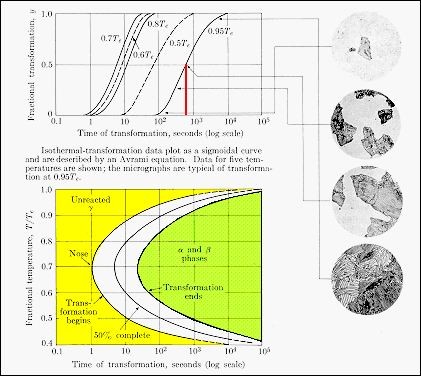Kinetics and Microstructural Control Isothermal Transformations · Following a phase transition as a function of time at selected transformation temperatures gives curves of the form shown.· A given transformation-time curve can be described by the Avrami equation: y = {1 - exp(-Ktn)}, where K, and n are constants· The transformation rate, r(T), can be characterized by the 50% transformation point t0.5: r(T) = (1/ t0.5 )From: Guy, "Elements of Physical Metallurgy." Addison Wesley (1974)